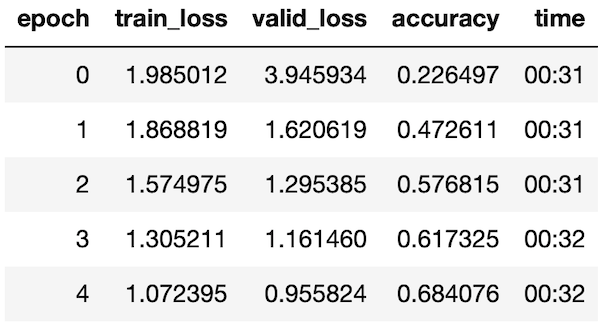# Faster Inference - Batch Normalization Folding

Hello folks !

Over the past few days I’ve tried to implement a simple yet efficient way to make inference faster without any change in performance for any model using Batch Normalization (i.e almost all of the recent ones) and I wanted to share it with you.

Note: I used fastai here but the code works for any PyTorch model

The intuition is this:

Batch Norm is very useful during the training phase as it allows the use of bigger learning rates and makes the training more stable, but at inference time, it is just used as a linear operation.

As a reminder, the output of the Batch Norm layer is :

y_{i}=\gamma \widehat{x}_{i}+\beta

\widehat{x}_{i} being the normalized input batch and \gamma, \beta being the parameters of the Batch Norm, learned during training.

And we know that the result of the combination of two linear operations is still a linear operation so there must be a way to combine the Batch Norm computation and the previous layer, a Convolution (what I tried for now).

So if I denote the convolution operation as:

z_i =W * x_i+b

Then, the output of Batch Norm can be re-written as:

y_i =\gamma \cdot \frac{z_i-\mu}{\sqrt{\sigma^{2}+\epsilon}}+\beta

So we can easily re-arrange the weights W and bias b of the convolution operation to take the Batch Norm into account, the result is:

\begin{aligned} w_{\text {fold }} &=\gamma \cdot \frac{W}{\sqrt{\sigma^{2}+\epsilon}} \\ b_{\text {fold }} &=\gamma \cdot \frac{b-\mu}{\sqrt{\sigma^{2}+\epsilon}}+\beta \end{aligned}

So if we swap the current W and b by the computed values above, we can safely remove the entire Batch Norm layer without changing anything in the results !

### How useful is it ?

Remove the Batch Norm layer should reduce the amount of parameters in the network (even though BN doesn’t have a lot) but more importantly it reduces the amount of computations to be done so should decrease the inference time!

I conducted some experiments on two commonly used architectures:

• VGG16 with BN
• Resnet50

Trained on ImageNette-160

#### VGG16

After training for 5 epochs (final accuracy doesn’t matter):The model has:

And inference takes:

After applying the BN folding technique:

We can first check that the performance is still the same:

And:

The gain in parameter is not huge as expected but there is some gain in inference time, of ~0.4ms.

#### Resnet50

The same process is applied to Resnet50:

Number of parameters:

Inference time:

After BN Folding:

So now, the drop in parameters is bigger (expected as unlike VGG, most of parameters are contained in convolutions), but look at that inference time gain ! 1.5ms !

### Code can be found here

This is a library I’m trying to build to make faster and lighter models with fastai. I’ll make another post explaining the other techniques used there.

If some of you are interested to collaborate or to help me to shift the code to fastai2.0, don’t hesitate to tell me !This is work in progress and I’m not a professional programmer so please excuse me for possible bugs or bad coding practice.

1 Like

@muellerzr I’ve seen your thread to make inference faster, maybe this might interest you